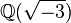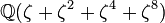# Linear representation theory of alternating groups

This article gives specific information, namely, linear representation theory, about a family of groups, namely: alternating group.
View linear representation theory of group families | View other specific information about alternating group

This article discusses the linear representation theory of the alternating group$A_n$ of finite degree$n$. Note that for$n = 0,1$, the alternating group coincides with the symmetric group, and for$n = 2$, it is trivial, so the interesting behavior begins from$n = 3$.

The article builds heavily on the linear representation theory of symmetric groups.

## Particular cases$n$ Alternating group Order Degrees of irreducible representations Number of irreducible representations Linear representation theory page
3 cyclic group:Z3 3 1,1,1 3 linear representation theory of cyclic group:Z3
4 alternating group:A4 12 1,1,1,3 4 linear representation theory of alternating group:A4
5 alternating group:A5 60 1,3,3,4,5 5 linear representation theory of alternating group:A5
6 alternating group:A6 360 1,5,5,8,8,9,10 7 linear representation theory of alternating group:A6
7 alternating group:A7 2520 1,6,10,10,14,14,15,21,35 9 linear representation theory of alternating group:A7
8 alternating group:A8 20160 1,7,14,20,21,21,21,28,35,45,45,56,64,70 14 linear representation theory of alternating group:A8
9 alternating group:A9 181440 [SHOW MORE] 18 linear representation theory of alternating group:A9
10 alternating group:A10 1814400 [SHOW MORE] 24 linear representation theory of alternating group:A10

## Combinatorics

### Split conjugacy classes

We have canonical bijections:

Conjugacy classes from$S_n$ that split in$A_n$$\leftrightarrow$ Partitions of$n$ into distinct odd parts (via splitting criterion)$\leftrightarrow$ Self-conjugate unordered integer partitions of$n$$\leftrightarrow$ Irreducible representations of$S_n$ that split in$A_n$

For more on these bijections, see set of self-conjugate unordered integer partitions

### Non-split conjugacy classes

We have equalities:

Number of conjugacy classes of even permutations in$S_n$ that don't split in$A_n$ = Number of conjugacy classes of odd permutations in$S_n$ = Number of (conjugate) pairs of non-self-conjugate partitions of$n$ = Number of pairs (in the sense of restricting to the same thing on$A_n$) of irreducible representations of$S_n$ that don't split in$A_n$.

### Totals

If$A$ equals the number of conjugacy classes of even permutations from$S_n$ that split in$A_n$, and$B$ equals the number of conjugacy classes of even permutations in$S_n$ that don't split i$A_n$, then:

• The number of conjugacy classes in$A_n$ is$2A + B$
• The number of conjugacy classes in$S_n$ is$A + 2B$$n$ Alternating group$A_n$ Order$A=$ number of irreducible representations from$S_n$ that split in$A_n$$B=$ number of irreducible representations from$S_n$ that don't split in$A_n$$2A + B =$ number of irreducible representations in$A_n$$A + 2B =$ number of irreducible representations in$S_n$$C =$ number of irreducible representations from$S_n$ which, even after splitting, remain real$2C + B =$ number of conjugacy classes of real elements in$A_n$$A + B + C=$ number of equivalence classes under real conjugacy in$A_n$
3 cyclic group:Z3 3 1 1 3 3 0 1 2
4 alternating group:A4 12 1 2 4 5 0 2 3
5 alternating group:A5 60 1 3 5 7 1 5 5
6 alternating group:A6 360 1 5 7 11 1 7 7
7 alternating group:A7 2520 1 7 9 15 0 7 8
8 alternating group:A8 20160 2 10 14 22 0 10 12
9 alternating group:A9 181440 2 14 18 30 1 16 17
10 alternating group:A10 1814400 2 20 24 42 2 24 24

## Splitting field

TERMINOLOGY AND FACTS TO CHECK AGAINST:
Terminology: field generated by character values (unique, cyclotomic) | minimal splitting field (not necessarily unique or cyclotomic) | splitting field | sufficiently large field (minimal choice is unique and cyclotomic)
Facts: sufficiently large implies splitting | splitting not implies sufficiently large | field generated by character values need not be a splitting field | field generated by character values is splitting field implies it is the unique minimal splitting field | minimal splitting field need not be unique | minimal splitting field need not be cyclotomic

### Field generated by character values

Below is information on the field generated by character values for the alternating group of degree$n$ for small values of$n$, all in characteristic zero. It is the same as the unique minimal splitting field, because all the irreducible representations have Schur index one.

Note that for each irreducible representation, the extension over the rationals for its character values, if nontrivial, is a quadratic extension. The overall field generated by character values is thus a Galois extension of the rationals with Galois group an elementary abelian 2-group and extension degree a power of 2.$n$ Alternating group$A_n$ Field generated by character values =unique minimal splitting field Degree of extension over rationals Smallest$m$ such that the cyclotomic extension with$m^{th}$ roots of unity contains the field generated by character values Degree of cyclotomic extension over rationals Galois group of extension over rationals
3 cyclic group:Z3$\mathbb{Q}[t]/(t^2 + t + 1)$, same as$\mathbb{Q}(\sqrt{-3})$ 2 3 2 cyclic group:Z2
4 alternating group:A4$\mathbb{Q}[t]/(t^2 + t + 1)$, same as$\mathbb{Q}(\sqrt{-3})$ 2 3 2 cyclic group:Z2
5 alternating group:A5$\mathbb{Q}(\cos(2\pi/5))$, same as$\mathbb{Q}(\sqrt{5})$ 2 5 4 cyclic group:Z2
6 alternating group:A6$\mathbb{Q}(\cos(2\pi/5))$, same as$\mathbb{Q}(\sqrt{5})$ 2 5 4 Klein four-group
7 alternating group:A7$\mathbb{Q}(\omega + \omega^2 + \omega^4)$ where$\omega$ is a primitive seventh root of unity
Same as$\mathbb{Q}(\sqrt{-7})$
2 7 6 cyclic group:Z2
8 alternating group:A8$\mathbb{Q}(\omega + \omega^2 + \omega^4, \zeta + \zeta^2 + \zeta^4 + \zeta^8)$ where$\omega$ is a primitive seventh root of unity and$\zeta$ is a primitive fifteenth root of unity
Same as$\mathbb{Q}(\sqrt{-7},\sqrt{-15})$
4 105 48 Klein four-group
9 alternating group:A9$\mathbb{Q}(\zeta + \zeta^2 + \zeta^4 + \zeta^8)$ where$\zeta$ is a primitive fifteenth root of unity
Same as$\mathbb{Q}(\sqrt{-15})$
2 15 8 cyclic group:Z2

### Criterion for the field generated by character values to be real

For a finite group, the field generated by character values is real if and only if the group is an ambivalent group, i.e., every element is conjugate to its inverse. For alternating groups, using the criterion for element of alternating group to be real, we can obtain a classification of ambivalent alternating groups, which basically gives us that the alternating group of degree$n$ is ambivalent iff$n \in \{ 1,2,5,6,10,14 \}$.